Monday 26th August 2019# Classroom Key Stage 1 / KS1 - Maths Activities & Resources

## Welcome to the Maths section of the Key Stage 1 classroom. Within KS1 Maths you will find resources, activities and online @school assessment tests that will help children revise and develop the subject of Maths at Key Stage 1 level

•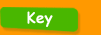•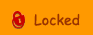•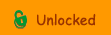••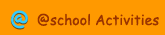•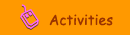•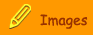The Activity you have selected is currently locked. Please register or Login to view.

###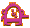Place Value and Number Order

Abacus Numbers - Interactive activity where you need to place the beads on the abacus to show the correct number.Before and After - Interactive activity to choose the number before and after a given number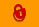Connect the Dots - Connect the dots and complete the picture.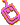Counting Crows - Interactive activity where you need to match up numbers to 10.Dienes Blocks - Interactive activity where you need to click and drag Dienes blocks to represent the correct numbers.Even Numbers - Test about odd and even numbers.Even Numbers Between - Interactive activity to find the even number between 2 numbersHow many Tens? - Interactive activity to say how many tens in a numberHundred Square - Interactive hundred squareIn Between - Interactive activity to select the number in between two numbers.Mend The Number Square - Interactive activity to find missing numbers from a number squareNumber - Work out what the highest number is on the number grid with this test.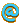Number Balance - Interactive activity about number propertiesNumber Grid to 20 - Interactive activity about number order up to 20.Number Lines - Interactive activity to identify the missing numbers on a numberlineNumber Lines - Interactive activity about counting on a number line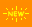Number Lines 2 - Interactive activity to identify the missing numbers on a numberlineNumber Order - An online activity to help ordering a set of 2 digit numbers.Number Order 1 - Interactive test to put a set of numbers into the correct order.Odd Numbers Between - Interactive activity to find the odd number between 2 numbersOdds - An interactive test to find odd numbers.One Less - Interactive activity to write the number that is one less than the given number.One Less  Year 2 - Interactive activity to write the number that is one less than the given number.One More - Interactive activity to identify the number that is one more.One More  Year 2 - Interactive activity to write the number that is one more than the given number.Order Numbers 1-100 - Interactive activity to order numbers 1-100Order Numbers 1-20 - Interactive activity to order numbers 1-20Park the Pods - Park the pods on the number square.Partitioning Numbers 1 - Interactive activity to practice partitioning of 2 digit numbers.Place the Penguins - Place the penguins on the number gridSubtract Ten - Interactive activity to subtract 10 from teen numbers.Ten Less - Interactive activity to say the number that is ten less than a given numberTen Less  Year 2 - Interactive activity to write the number that is ten less than the given number.Ten More - Interactive activity to identify the number that is ten more.Ten More  Year 2 - Interactive activity to write the number that is ten more than the given number.Ten More Ten Less - Interactive activity to identify numbers that are 10 more and 10 less than a given numberTens and Units - Interactive activity to say how many tens and units in the numbers given.The Biggest Number - Interactive activity to identify the biggest number.Wash Line - Put the numbers in orderWhich has more? - Interactive activity to choose the picture with the most animals.Who Is First? - Interactive activity to identify positions in a queue.